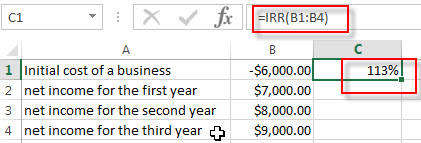# Excel IRR Function

This post will guide you how to use Excel IRR function with syntax and examples in Microsoft excel.

### Description

The Excel IRR function returns the internal rate of return for a series of cash flows and the cash flows must be occurred at regular intervals (monthly or annually).

The internal rate of return is the interest rate received for an investment consisting of payments (negative values) and income (positive values) that occur at regular periods.

The IRR function is a build-in function in Microsoft Excel and it is categorized as a Financial Function.

The IRR function is available in Excel 2016, Excel 2013, Excel 2010, Excel 2007, Excel 2011 for Mac.

### Syntax

The syntax of the IRR function is as below:

`=IRR(values, [guess])`

Where the IRR function arguments are:

• Values -This is a required argument. An array or cell reference that contain numbers for which you want to calculate the internal rate of return. And the values must include at least one positive value and one negative value.
• Guess – This is an optional argument. An initial guess at the internal rate of return. If it is omitted, and it will be set as 0.1 or 10%.

Note:

• IRR is closely related to NPV, the net present value function. The rate of return calculated by IRR is the interest rate corresponding to a 0 (zero) net present value. The following formula demonstrates how NPV and IRR are related:
• NPV(IRR(A2:A7),A2:A7) equals 1.79E-09 [Within the accuracy of the IRR calculation, the value is effectively 0 (zero).]

### Excel IRR Function Examples

The below examples will show you how to use Excel IRR Function to calculate the internal rate of return for a series of cash flows.

#1 to get the investment’s internal rate of return after 3 years, using the following formula:

`=IRR(B1:B4)`### Related Functions

• Excel FV Function
The Excel FV function used to calculate the future value of an investment based on a constant interest rate. The syntax of the FV function is as below:=FV(rate,nper,pmt,[pv],[type])…
• Excel IPMT Function
The Excel IPMT function used to calculate the interest payment for an investment based on a constant payment schedule and a constant interest rage.The syntax of the IPMT function is as below:= IPMT (rate, per, nper, pv, [fv], [type])…
• Excel ISPMT Function
The Excel ISPMT function used to calculate the interest paid during a specific period of an investment.The syntax of the ISPMT function is as below:= ISPMT (rate, per, nper, pv)…
• Excel MIRR Function
The Excel MIRR function returns the modified internal rate of return for a series of cash flows and the cash flows must be occurred at regular intervals (monthly or annually). The syntax of the MIRR function is as below:=MIRR(values, finance_rate,reinvest_rate)…
Excel NPER Function
The Excel NPER function returns the number of periods for an investment or loan based on periodic payment amount and a constant interest rate.The syntax of the NPER function is as below:= NPER (rate, pmt, pv, [fv], [type])…
• Excel NPV Function
The Excel NPV function returns the net present value of an investment by using a discount rate and a series of future cash flows (future payments and income).The syntax of the NPV function is as below:= NPV (rate, Value1,[value2],…)…
• Excel PMT Function
The Excel PMT function returns the payment amount for a loan or investment based on constant payments and a constant interest rate.The syntax of the PMT function is as below:= PMT(rate, nper, pv,[fv],[type])…
• Excel PPMT Function
The Excel PPMT function returns the payment amount on the principal for a given period for a loan or investment based on constant payments and a constant interest rate. The syntax of the PPMT function is as below:=PPMT(rate, per,nper, pv,[fv],[type])…
• Excel RATE Function
The Excel RATE function returns the interest rate per payment period of an annuity.The syntax of the RATE function is as below:=RATE(nper, pmt,pv,[fv],[type],[guess])…
• Excel PV Function
The Excel PV function returns the present value of a loan or investment based on constant payments and a constant interest rate. So you can use the PV function to get the present value based on a series of future payments.The syntax of the PV function is as below:= PV(rate,nper,pmt,[fv],[type])…